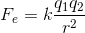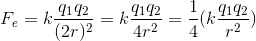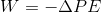# SAT II Physics : Electric Fields

## Example Questions

### Example Question #1 : Coulomb's Law

If the distance between two charged particles is doubled, the strength of the electric force between them will __________.

be quartered

remain unchanged

be halved

double

be quartered

Explanation:

Coulomb's law gives the relationship between the force of an electric field and the distance between two charges:The strength of the force will be inversely proportional to the square of the distance between the charges.

When the distance between the charges is doubled, the total force will be divided by four (quartered).### Example Question #1 : Electricity And Magnetism

On which of the following does the amount of work required to move a charge in an electric field depend?

Only the change in potential

Only the magnetic field

Both the potential and the path traveled

Neither the potential nor the path traveled

Only the path traveled

Only the change in potential

Explanation:

Work done by electric field is defined:Notice that the only variable in the equation is the potential, so this is the only quantity on which work depends.

### All SAT II Physics Resources